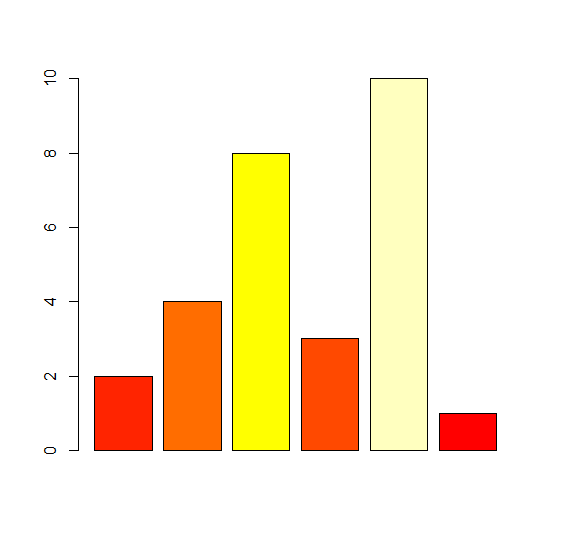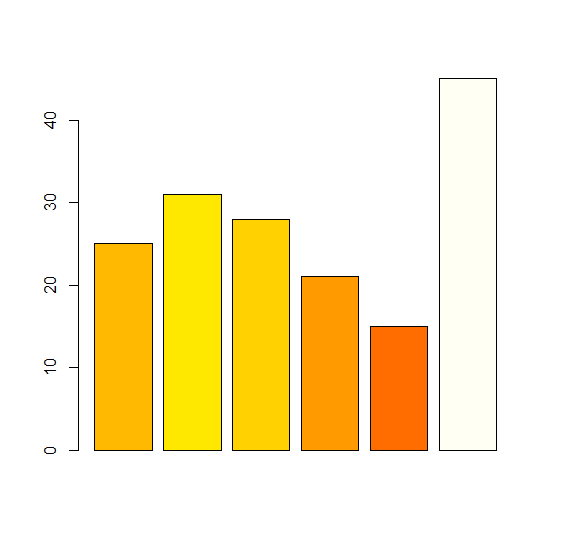# Fill bars in a base R barplot with colors based on frequency.

Suppose we have a vector that contains only frequencies and we want to create a bar chart in base R using these frequencies with color of bars based on frequencies, therefore, we can use barplot function and providing the color of the bars with as shown in the below Examples

The function is as follows −

heat.colors function

## Example 1

To fill the bars in a base R barplot with colours based on frequency, use the command given below −

Frequency_1<-c(2,4,8,3,10,1)
barplot(Frequency_1,col=heat.colors(max(Frequency_1))[Frequency_1])

## Output

If you execute the above given snippet, it generates the following Output −## Example 2

To fill the bars in a base R barplot with colours based on frequency, use the command given below −

Frequency_2<-c(25,31,28,21,15,45)
barplot(Frequency_2,col=heat.colors(max(Frequency_2))[Frequency_2])

## Output

If you execute the above given snippet, it generates the following Output −